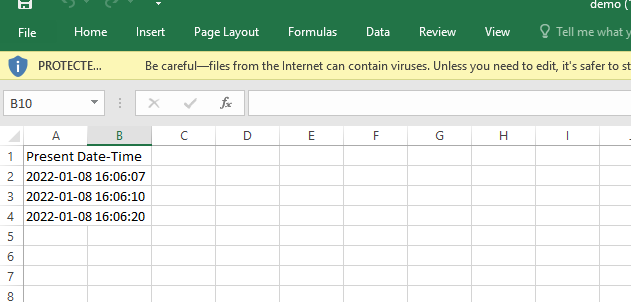# How to add timestamp to excel file in Python

Modules Needed:

1) datetime: In Python, this module allows us to work with dates and times.

Installation

`pip install datetime`

2)openpyxl: This is a Python library that allows you to read and write Excel files.

Installation

`pip install openpyxl`

3)time: The time module provides a wide variety of time-related functions.

strftime() Method:

Date and time objects are converted to string representations using the strftime() method. It takes one or more formatted code inputs and returns the string representation.

## How to Add Timestamp to Excel File in Python

Example

Approach:

• Import datetime module using the import keyword.
• Import Workbook from openpyxl module using the import keyword from openpyxl import Workbook.
• Import time module using the import keyword.
• Create a workbook object and store it in a variable.
• Choose the active sheet and store it in another variable.
• Change the heading of the cell A1(rowno-1 and colno-1) to some random text.
• Get the present date and time using datetime.now() function and modify the cell A2(rowno-2 and colno-1) value to the Current Date and Time using value.
• Apply sleep() method by passing some random number as an argument to it to sleep it for the given n seconds.
• Get the present date and time using datetime.now() function and modify the cell A3(rowno-3 and colno-1) value to the Current Date and Time using value.
• Apply sleep() method by passing some random number as an argument to it to sleep it for the given n seconds.
• Get the present date and time using datetime.now() function and modify the cell A4(rowno-4 and colno-1) value to the Current Date and Time using value.
• Pass some random filename as an argument to the save() function to save the above workbook.
• Close the workbook using the close() function.
• The Exit of the Program.

Below is the implementation:

```# Import datetime module using the import keyword
import datetime
# Import Workbook from openpyxl module using the import keyword
from openpyxl import Workbook
# Import time module using the import keyword
import time
# Create a workbook object and store it in a variable
work_book = Workbook()
# Choose the active sheet using active and store it in another variable
work_sheet = work_book.active
# Change the heading of the cell A1(rowno-1 and colno-1) to some random text
work_sheet.cell(row=1, column=1).value = "Present Date-Time"

# Get the present date and time using datetime.now() function and modify the cell
# A2(rowno-2 and colno-1) value to the Current Date and Time using value.
work_sheet.cell(row=2, column=1).value = datetime.datetime.now().strftime('%Y-%m-%d %H:%M:%S')

# Apply sleep() method by passing some random number as an argument to it
# to sleep it for the given n seconds.
time.sleep(3)
# Get the present date and time using datetime.now() function and modify the cell
# A3(rowno-3 and colno-1) value to the Current Date and Time using value.
work_sheet.cell(row=3, column=1).value = datetime.datetime.now().strftime('%Y-%m-%d %H:%M:%S')
time.sleep(10)
# Get the present date and time using datetime.now() function and modify the cell
# A4(rowno-4 and colno-1) value to the Current Date and Time using value.
work_sheet.cell(row=4, column=1).value = datetime.datetime.now().strftime('%Y-%m-%d %H:%M:%S')

# Pass some random filename as an argument to the save() function
# to save the above workbook.
work_book.save('demo.xlsx')
# Close the workbook using the close() function
work_book.close()```

Output: